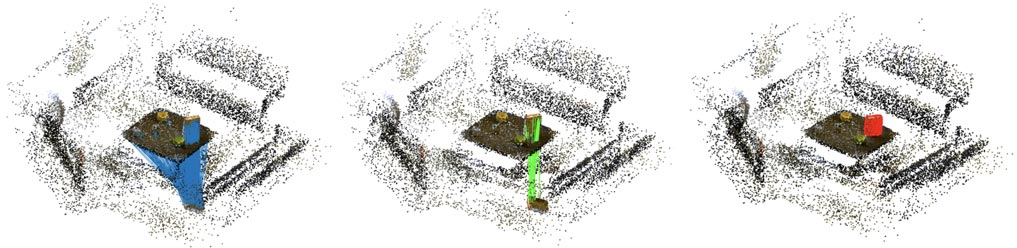}

# Polynomial-time Robust Registration with Extreme Outlier RatesPoint cloud registration is a crucial problem in robotics and computer vision, with extensive applications such as object detection and localization and motion estimation and 3D reconstruction. In order to register two point clouds, it is popular to first extract and match feature points to establish correspondences, followed by solving an optimization problem to find the best rigid transformation (scale, rotation and translation).

In practice, a large amount of the correspondences can be wrong, therefore, it is of paramount importance to develop a robust optimization backend that can tolerate extreme amount of outliers. We introduce TEASER (Truncated least squares Estimation And SEmidefinite Relaxation) (Yang & Carlone, 2019) , the first polynomial time algorithm that is certifiably optimal and robust against 99% outliers. We propose a robust approach for the registration of two sets of 3D points in the presence of a large amount of outliers.

There are three main contribution in this work:

1. we reformulate the registration problem using a Truncated Least Squares (TLS) cost that makes the estimation insensitive to a large fraction of spurious point-to-point correspondences
2. we develop a general framework to decouple rotation, translation, and scale estimation, which allows solving in cascade for the three transformations.
3. Since each subproblem (scale, rotation, and translation estimation) is still non-convex and combinatorial in nature, we show that
• TLS scale and (component-wise) translation estimation can be solved exactly and in polynomial time via an adaptive voting scheme,
• TLS rotation estimation can be relaxed to a semidefinite program and the relaxation is tight (exact) in practice, even in presence of an extreme amount of outliers.We validate TEASER using standard registration benchmarks showing that the algorithm outperforms RANSAC and robust local optimization techniques, and favorably compares with Branch-and-Bound methods, while being a polynomial-time algorithm.

### Video presentation

1. Yang, H., & Carlone, L. (2019). A Polynomial-time Solution for Robust Registration with Extreme Outlier Rates.